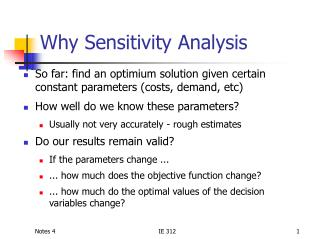Download PresentationWhy Sensitivity Analysis

# Why Sensitivity Analysis - PowerPoint PPT PresentationDownload Presentation## Why Sensitivity Analysis

- - - - - - - - - - - - - - - - - - - - - - - - - - - E N D - - - - - - - - - - - - - - - - - - - - - - - - - - -
##### Presentation Transcript

1. Why Sensitivity Analysis • So far: find an optimium solution given certain constant parameters (costs, demand, etc) • How well do we know these parameters? • Usually not very accurately - rough estimates • Do our results remain valid? • If the parameters change ... • ... how much does the objective function change? • ... how much do the optimal values of the decision variables change? IE 312

2. General Optimization Problem • Minimize some cost or maximize benefit • Constraints: • £ Restrictions on supply of some resource • ³ Restriction on satisfying demand for some resource • = Both supply restriction and demand requirement • Variable-type constraints • Decision variable determine the levels of some activity • Coefficients = per unit impact of activities IE 312

3. Changing Constraints • Relaxing constraints: • Optimal value same or better • Tightening constraints: • Optimal value same or worse Original Relaxed Tightened IE 312

4. Crude Oil Model Satisfy Demand Supply Restriction IE 312

5. Solution (LINDO) LP OPTIMUM FOUND AT STEP 1 OBJECTIVE FUNCTION VALUE 1) 112.5000 VARIABLE VALUE REDUCED COST X1 2.000000 0.000000 X2 3.500000 0.000000 ROW SLACK OR SURPLUS DUAL PRICES 2) 0.000000 -37.500000 3) 0.000000 0.000000 4) 0.950000 0.000000 5) 7.000000 0.000000 6) 2.500000 0.000000 7) 0.000000 -18.750000 8) 1.500000 0.000000 IE 312

6. Sensitivity ‘Plenty’ of this crude IE 312

7. RHS Coefficients IE 312

8. LHS Coefficients IE 312

9. New Constraints • Adding constraints tightens the feasible set • Removing constraints relaxes the feasible set • What about unmodeled constraints? IE 312

10. Optimal Value Optimal Value Optimal Value Optimal Value RHS RHS RHS RHS Rate of Change Supply Demand Maximize Minimize IE 312

11. Rate of Change Maximize Minimize Optimal Value Optimal Value Coefficient. Coefficient. IE 312

12. New Activities • Adding activities • Optimal value same or better • Removing activities • Optimal value same or worse IE 312

13. Quantifying Effects • Now know the qualitative effects of • Changing RHS coefficients • Changing LHS coefficients • Changing objective function coefficients • Adding/deleting constraints • Adding/deleting activities • How much does the objective change? • Quantitative change IE 312

14. Back to Crude Oil Example Decreasing RHS will make objective better or no worse, but by how much? How much are we willing to pay to have one more barrel available? IE 312

15. Answer using the Dual IE 312

16. LINDO Solution LP OPTIMUM FOUND AT STEP 4 OBJECTIVE FUNCTION VALUE 1) 92.50000 VARIABLE VALUE REDUCED COST V1 20.000000 0.000000 V2 35.000000 0.000000 V3 0.000000 0.950000 V4 0.000000 0.000000 V5 0.000000 0.000000 IE 312

17. Interpretation • Our cost will be reduced by \$20 or \$ 35, respectively, if the demand for gasoline or jet fuel is one unit less. • Smaller demand for lubricants has no effect on the objective • We are not willing to pay anything for availability of more crude! IE 312

18. What is the Dual? • The primal is the original optimization problem • The dual is an LP defined on the same input parameters but characterizing the sensitivities of the primal • There is one dual variable for each main constraint IE 312

19. Interpretation • The dual variables provide implicit prices for marginal units of the resource modeled by the constraint • Zero unless active • How much we are willing to pay for more of a resource (supply constraint) • How much we benefit from not having to satisfy a requirement (demand constraint) IE 312

20. What to Optimize? • Implicit marginal value (minimization primal) or price (maximization primal) is • Maximize value or minimize price! IE 312

21. Dual Constraints • For each activity xj in a minimization primal there is a main dual constraint • For a maximization primal, each xj 0 has a main dual constraint IE 312

22. Optimal Solution • If primal has optimal solution • Either the primal optimal makes a main inequality active or the corresponding dual is zero • Either a nonnegative primal variable has optimal value xj= 0 or the corresponding dual price vj must make the j-th dual constraint active IE 312

23. Dual of a Min Primal IE 312

24. Dual of a Max Primal IE 312

25. Top Brass IE 312

26. 2000 1500 1000 500 500 1000 1500 2000 Graphical Solution Optimal Solution IE 312

27. Dual IE 312

28. Lindo Solution OBJECTIVE FUNCTION VALUE 1) 17700.00 VARIABLE VALUE REDUCED COST V1 0.000000 350.000000 V2 0.000000 400.000000 V3 6.000000 0.000000 V4 1.500000 0.000000 IE 312

29. Interpretation • We are willing to pay up to \$6/each for additional brass plaques • We are willing to pay up to \$1.5/foot for more wood • We don’t need any more brass footballs or soccer balls Our objective is sensitive to these estimates! IE 312

30. Formulating Duals IE 312

31. Formulating Duals IE 312